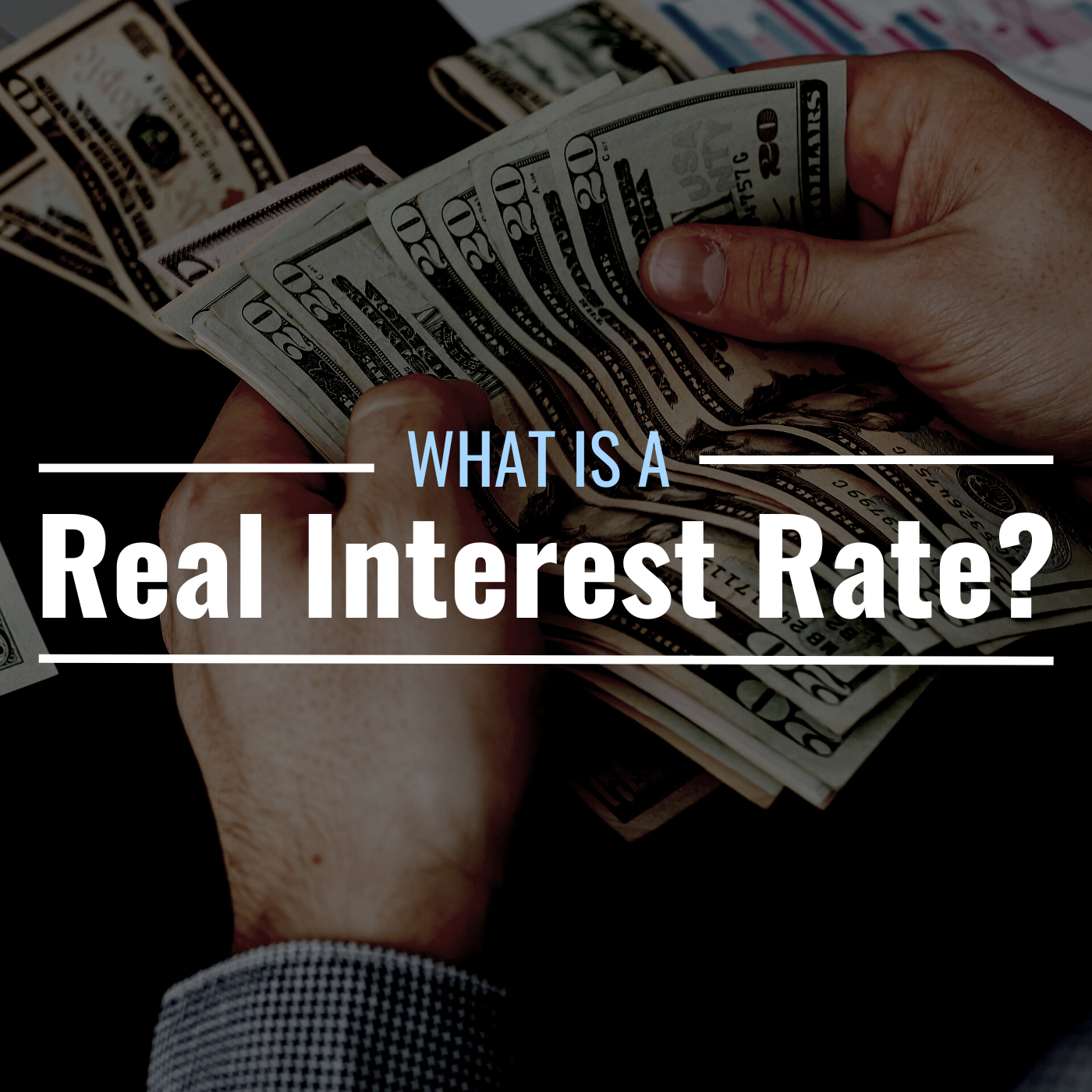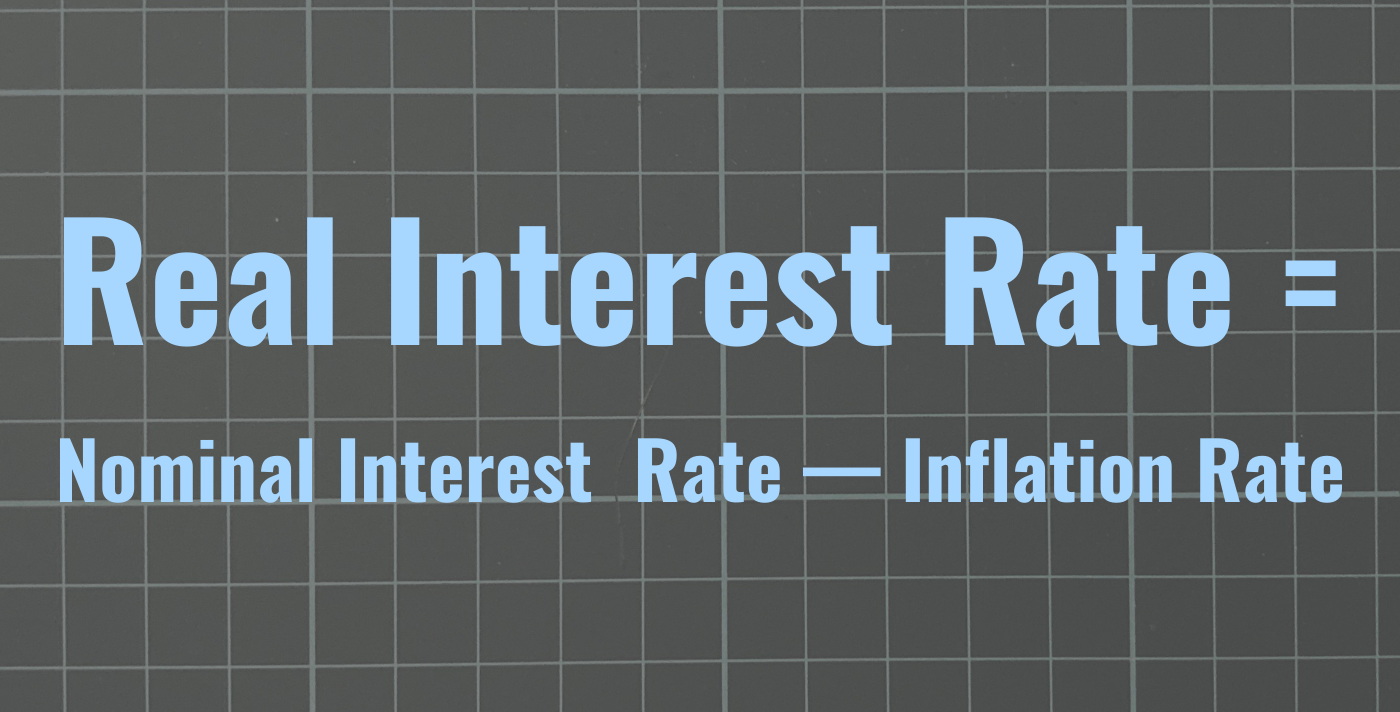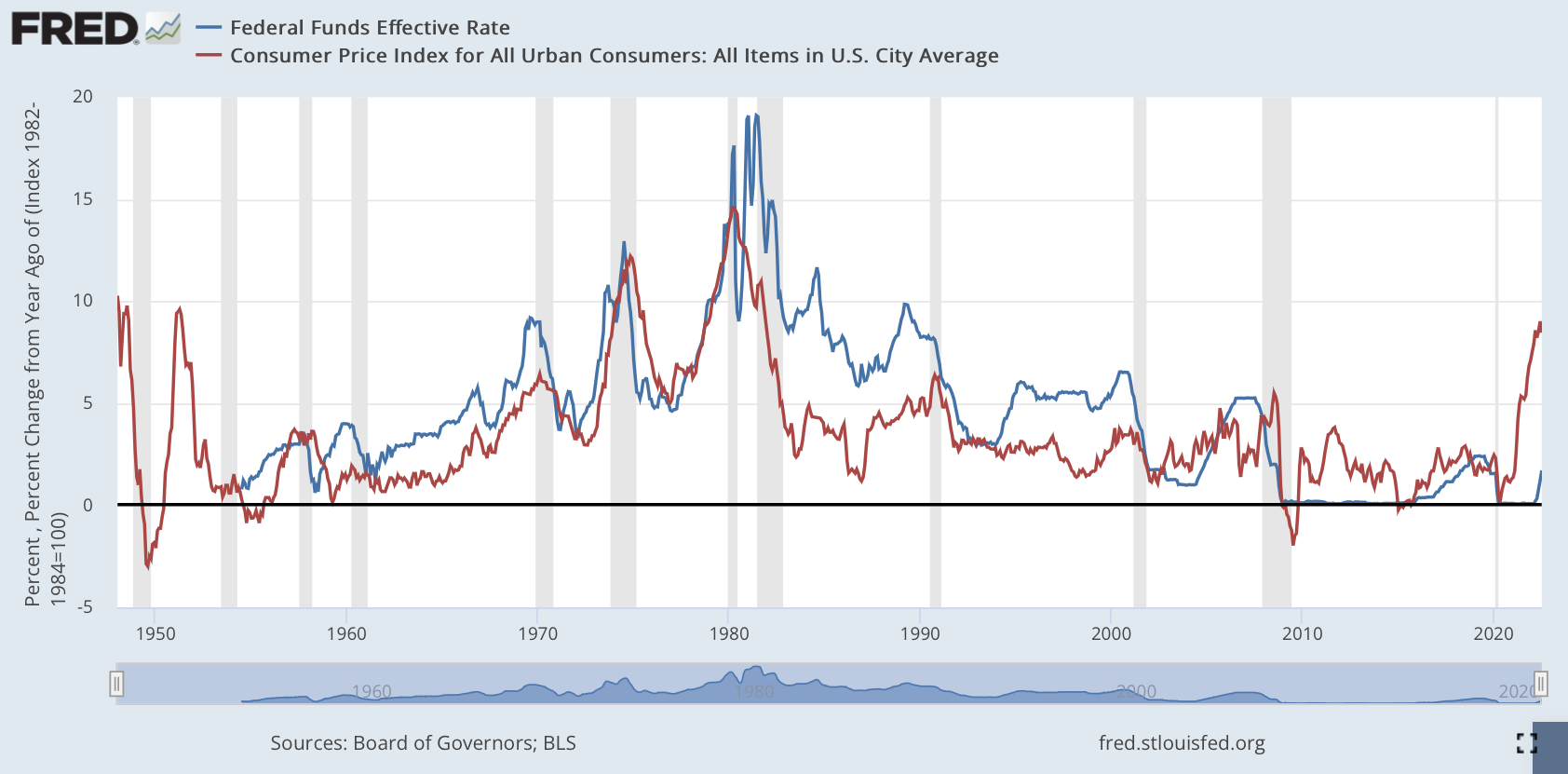# What is a real interest rate? Definition and what it means to you

## What is a real interest rate?

A real interest rate is the difference between a nominal interest rate and the rate of inflation.

Nominal interest rates are the rates set by financial institutions, such as banks and credit card companies, to be paid as interest on borrowed money. These are also the rates at which money can be earned from a savings account, bond, or certificate of deposit.

Money earned or paid on interest, however, can be misleading because the rate of inflation is not taken into account. A high inflation rate can erode purchasing power and, at the same time, reduce the value of debt payments in real terms, which is why the real interest rate gives a better picture of one or the other.

## Examples of Real Interest Rates

For example, putting \$1,000 in a savings account that pays 2% annual interest at the start of the year would earn \$1,020 at the end of the year. But with an annual inflation rate – based on the consumer price index – of 10%, there would be no interest earned, and the “real” value of savings would erode by 8 %, which means that the deposit (in real terms) will be valued at \$920 adjusted for inflation by the end of the year.

If, however, the savings rate were 10% and the inflation rate 2%, the real interest rate would be 8%, meaning the savings account would be valued at \$1,080 adjusted inflation by the end of the year.

The same goes for interest rates on debt. A borrower would benefit from a higher inflation rate if the interest rate were lower. Yet, the bank issuing the debt would be on the losing side as it would earn no interest in real terms.

This would be especially the case if the rates were fixed. However, if loan terms are not fixed, banks can change interest rates, as with adjustable rate mortgages (ARMs), to account for changes in the rate of inflation.

Scroll to continue

## How to calculate the real interest rate

The simplest way to calculate the real interest rate is to subtract the inflation rate from the nominal interest rate.Real interest rate = Nominal interest rate – Inflation rate Cloth

## How does monetary policy affect real interest rates?

The Federal Reserve can influence the direction of the economy with its monetary policy. High inflation may prompt the central bank to tighten monetary policy, while low inflation may prompt looser policy.

Below is a graph comparing the effective federal funds rate (which is the weighted average rate that borrowing institutions pay lending institutions) to the consumer price index from July 1954 to July 2022. The effective rate , represented by the blue line, has generally been above the rate of inflation, in red. Real interest rates have been positive most of the time, except for certain periods, such as the mid-1970s and early 2000s.The federal funds rate has always been higher than the rate of inflation. Screenshot via Federal Reserve Bank of St. Louis

In the aftermath of the 2007-2008 financial crisis, the Fed followed a zero interest rate policy from December 2008 to December 2015 to stimulate economic growth. Inflation tended to remain below 2% during this period, and the target range for the federal funds rate was set between 0 and 0.25%.

The Fed slowly began raising interest rates to keep up with inflation starting in 2016. At the onset of the COVID-19 pandemic in 2020, however, rates were lowered to near zero to aid recovery economic. But as inflation began to rise in 2021, the Fed lagged in its response with rate hikes.

The chart shows that the Fed’s aggressive monetary policy (in the form of big rate hikes) tends to precede recessions, which are highlighted by shaded areas.

## Can the real interest rate be negative?

If the inflation rate exceeds the nominal interest rate, then the real interest rate becomes negative. Negative real interest rates are bad for savers because (in purchasing power terms) they don’t actually earn interest on their deposits when adjusted for inflation. It’s also bad for banks because inflation reduces the real return on a loan.